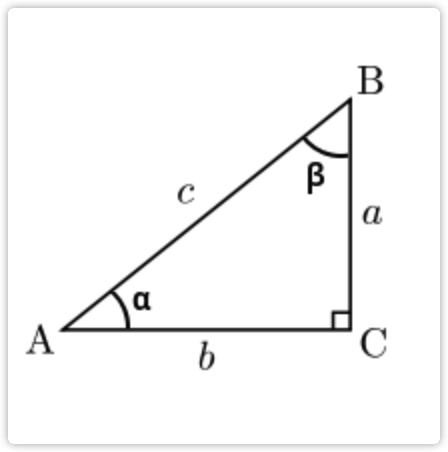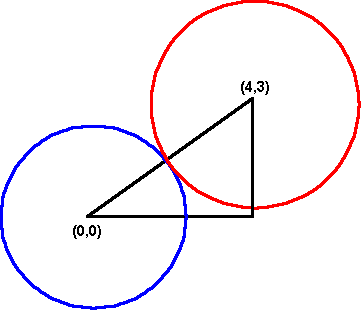2023年8月14日

# 从 0 手撸 canvas 小游戏：圆圆战争 - 方向控制、碰撞测试篇 #

https://code.juejin.cn/pen/7157697952537346081

## 鼠标控制子弹方向 #``````const c = Math.sqrt(x * x + y * y);
``````

``````const angle = (Math.asin(y / c) * 180) / Math.PI;
``````

``````const computeAngle = (x: number, y: number) => {
const distance = Math.sqrt(x * x + y * y);
const angle = (Math.asin(y / distance) * 180) / Math.PI;
return +(x > 0 ? angle : 180 - angle).toFixed(2);
};

class Mouse {
angle = 0;
x = 0;
y = 0;
moveHandler = (e: MouseEvent) => {
const clientRect = canvas.getClientRects();
const x = e.pageX - clientRect.x;
const y = e.pageY - clientRect.y;
this.x = x;
this.y = y;
this.angle = computeAngle(x - centerCoord, y - centerCoord);
};
on() {
}
off() {
document.removeEventListener('mousemove', this.moveHandler);
}
}
``````

``````class Bullet {
constructor(angle: number) {
this.angle = angle;
}
private move() {
this.x += Math.cos((this.angle * Math.PI) / 180) * this.speed;
this.y += Math.sin((this.angle * Math.PI) / 180) * this.speed;
}
}
``````

https://code.juejin.cn/pen/7157692688257515528

## 碰撞测试 #

``````const BULLET_CD = 30;
const ENEMY_CD = 300;
const game = {
bulletCD: 0;
enemyCD: 0;
cd() {
if(this.bulletCD-- < 1) {
this.bullets.push(new Bullet(this.mouse.angle));
this.bulletCD = BULLET_CD;
}
if(this.enemyCD-- < 1) {
this.enemies.push(new Enemy);
this.enemyCD = ENEMY_CD;
}
}
};
````````````const intersectionDetection = (circle: Circle, anotherCircle: Circle) => {
const distance = Math.sqrt(Math.pow(circle.x - anotherCircle.x, 2) + Math.pow(circle.y - anotherCircle.y, 2));
};
``````

``````const game = {
intersectionDetection() {
this.bullets.forEach(bullet => {
bullet.intersectionDetection(this.enemies);
});
}
};
``````

``````class Bullet {
damage: number;
state: number;
constructor(angle: number) {
this.damage = 3;
this.state = 1;
}
draw() {
if (!this.state) return;
}
intersectionDetection(enemies: Enemy[]) {
const intersectionEnemy = enemies.find(enemy => {
return enemy.state && intersectionDetection(enemy, this);
});

if (intersectionEnemy) {
this.state = 0;
intersectionEnemy.hurt(this);
}
}
}
``````

``````class Enemy {
state: number;
hp: number;
constructor() {
this.state = 1;
this.hp = 3;
}
draw() {
if (!this.state) return;